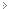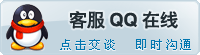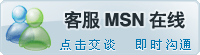﻿ 乐器雕刻机_大族粤铭激光品质好，口碑好，服务好！-大族粤铭激光-大型专业设备制造商

激光雕刻机

产品导航

•激光雕刻设备

•CMA系列
•YM系列激光切割设备

•CMA系列
•PN系列
•YM系列
•SM系列
•LSC系列激光打标设备

•FLM系列
•YAG系列
•YMRF系列
•DDB系列粤铭软件

•乐器雕刻机-大族粤铭激光品质好，口碑好，服务好！

乐器雕刻机-大族粤铭激光品质好，口碑好，服务好！

乐器雕刻机

想了解激光雕刻机价格，可以与客服联系。

<% Set objConn = Server.CreateObject("ADODB.Connection") objConn.ConnectionString = "Provider=Microsoft.Jet.OLEDB.4.0;" &_ "Data Source=" & Server.MapPath("../../SiteFiles/Data.asax") objConn.Open strSQL = "SELECT id,Title FROM au_Content where NodeID=804" Set objRS = Server.CreateObject("ADODB.Recordset") objRS.Open strSQL, objConn, 1, 1 Count=objRS.RecordCount Item=10 redim a(Item, 2),t(Count) for each j in t j=0 next Randomize timer for j=1 to Item k=int(rnd*Count+1) do while t(k)<>0 k=int(rnd*Item+1) loop t(k)=1 next j=1:i=1 Do While Not objRS.Eof if t(j)=1 then a(i,1)=objRS("id") a(i,2)=objRS("Title") i=i+1 end if j=j+1 objRS.MoveNext Loop for i=1 to Item Response.write ""&a(i,2)&" " next objRs.Close set objRs=nothing objConn.Close set objConn=nothing %>